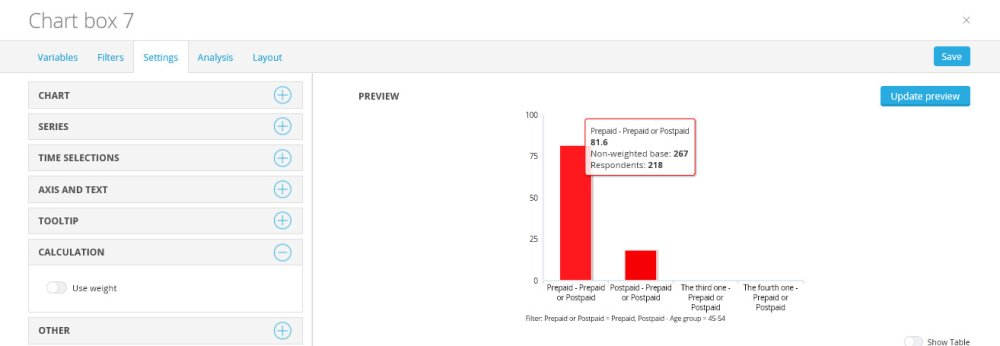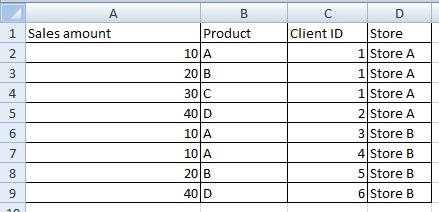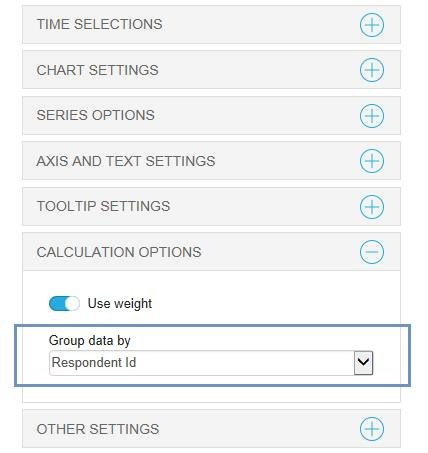The chart calculation menu contains the option for showing weighted or unweighted data in the chart by selecting the button as showing in the example. Here is the example of the chart with tooltip showing unweighted chart data.The calculation menu also contains a Group data drop down menu. The calculation setting present under this menu is used for handling data stored as transactions which makes it possible to calculate mean values of a respondent where data is stored in multiple rows in the imported data file. The example data file below shows how a transnational formatted data file could look like.The file shows sales data from a store. When a client buys multiple items the client is shown as one row per item in the data file. For example if row 1-3 belong to the same client, column C shows a “client ID” which makes it possible to find out which of the rows that belongs to the same client. When reporting this data file one measurement could be the “Average sales per client”.

By using the original “Numeric mean value” calculation in Dapresy Pro a mean value is calculated based on all rows in the data file and not based on all the clients which had been given an incorrect result. Due to that a new “Group Data by” function has been added with the purpose to calculate mean values of transaction data correctly. The table below shows a typical transaction formatted data file.

When using “Group data by” the mean value is calculated by the grouped respondents and not per respondent. The example below explains the calculation. The table above shows transaction formatted sales data. To get the “Average sales per client” the Sales Amount variable is used when creating a chart or table. By using the original calculation logic the average sales per client for Store A is based by the number of rows which gives the result (10+20+30+40)/4= 25 which is wrong in this particular case as the wanted result is the average sales per client and not per row.

By using the “Group data by” option and selecting to group the data by the “Client ID” variable the mean value is based by the number of clients which gives the result (10+20+30+40)/2=50 which is correct in this particular case.

A control for selecting which variable to group the data by is present in the Chart setup in the Settings tab as shown in the image below. The control does only appear when Numeric variables are selected in the variable list. The variable selected in the “Group data by” list is used to group the data during the calculation. The default variable in the list is the “Respondent ID” which means that no grouping is made as each respondent has a unique id. The image below shows the new “Group data by:” selection list.